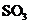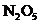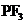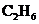Chapter 18.2, Problem 18.2SC### Introductory Chemistry: A Foundati...

9th Edition
Steven S. Zumdahl + 1 other
ISBN: 9781337399425

#### Solutions

Chapter
Section### Introductory Chemistry: A Foundati...

9th Edition
Steven S. Zumdahl + 1 other
ISBN: 9781337399425
Textbook Problem
5 views

# Exercise 18.2 Assign oxidation states to all atoms in the following molecules or ions.a.b.c.d.e.Interpretation Introduction

(a)

Interpretation:

The oxidation state of all atoms in SO3 should be assigned.

Concept Introduction:

The chemical reaction in which both oxidation and reduction process takes place is known as redox reaction. In this reaction, transfer of electrons takes place among the elements.

Oxidation is the process in which either loss of electrons, oxidation number increases, or loss of hydrogen atoms takes place. An element is oxidized, when oxidation number increases.

Reduction is the process in which either gain of electrons, oxidation number decreases, or gain of hydrogen atoms takes place. An element is reduced, when oxidation number decreases.

Oxidation state is also known as oxidation number. It is defined as the numbers which are assign to the elements in a chemical combination and number represents the electrons which an atom can share, lose or gain to form chemical bonding with an atom of another element.

Therefore, transfer of electrons refers to the oxidation state.

Explanation

The given molecule is:

SO3

According to the rules of oxidation states, oxygen is assigned as oxidation state of -2 in covalent compounds. Now, oxidation state of sulfur should be determined, since overall charge on the molecule is zero.

Therefore, let x be the oxidation state of sulfur

Interpretation Introduction

(b)

Interpretation:

The oxidation state of all atoms in SO42 should be assigned.

Concept Introduction:

The chemical reaction in which both oxidation and reduction process takes place is known as redox reaction. In this reaction, transfer of electrons takes place among the elements.

Oxidation is the process in which either loss of electrons, oxidation number increases, or loss of hydrogen atoms takes place. An element is oxidized, when oxidation number increases.

Reduction is the process in which either gain of electrons, oxidation number decreases, or gain of hydrogen atoms takes place. An element is reduced, when oxidation number decreases.

Oxidation state is also known as oxidation number. It is defined as the numbers which are assign to the elements in a chemical combination and number represents the electrons which an atom can share, lose or gain to form chemical bonding with an atom of another element.

Therefore, transfer of electrons refers to the oxidation state.

Interpretation Introduction

(c)

Interpretation:

The oxidation state of all atoms in N2O5 should be assigned.

Concept Introduction:

The chemical reaction in which both oxidation and reduction process takes place is known as redox reaction. In this reaction, transfer of electrons takes place among the elements.

Oxidation is the process in which either loss of electrons, oxidation number increases, or loss of hydrogen atoms takes place. An element is oxidized, when oxidation number increases.

Reduction is the process in which either gain of electrons, oxidation number decreases, or gain of hydrogen atoms takes place. An element is reduced, when oxidation number decreases.

Oxidation state is also known as oxidation number. It is defined as the numbers which are assign to the elements in a chemical combination and number represents the electrons which an atom can share, lose or gain to form chemical bonding with an atom of another element.

Therefore, transfer of electrons refers to the oxidation state.

Interpretation Introduction

(d)

Interpretation:

The oxidation state of all atoms in PF3 should be assigned.

Concept Introduction:

The chemical reaction in which both oxidation and reduction process takes place is known as redox reaction. In this reaction, transfer of electrons takes place among the elements.

Oxidation is the process in which either loss of electrons, oxidation number increases, or loss of hydrogen atoms takes place. An element is oxidized, when oxidation number increases.

Reduction is the process in which either gain of electrons, oxidation number decreases, or gain of hydrogen atoms takes place. An element is reduced, when oxidation number decreases.

Oxidation state is also known as oxidation number. It is defined as the numbers which are assign to the elements in a chemical combination and number represents the electrons which an atom can share, lose or gain to form chemical bonding with an atom of another element.

Therefore, transfer of electrons refers to the oxidation state.

Interpretation Introduction

(d)

Interpretation:

The oxidation state of all atoms in C2H6 should be assigned.

Concept Introduction:

The chemical reaction in which both oxidation and reduction process takes place is known as redox reaction. In this reaction, transfer of electrons takes place among the elements.

Oxidation is the process in which either loss of electrons, oxidation number increases, or loss of hydrogen atoms takes place. An element is oxidized, when oxidation number increases.

Reduction is the process in which either gain of electrons, oxidation number decreases, or gain of hydrogen atoms takes place. An element is reduced, when oxidation number decreases.

Oxidation state is also known as oxidation number. It is defined as the numbers which are assign to the elements in a chemical combination and number represents the electrons which an atom can share, lose or gain to form chemical bonding with an atom of another element.

Therefore, transfer of electrons refers to the oxidation state.

### Still sussing out bartleby?

Check out a sample textbook solution.

See a sample solution

#### The Solution to Your Study Problems

Bartleby provides explanations to thousands of textbook problems written by our experts, many with advanced degrees!

Get Started